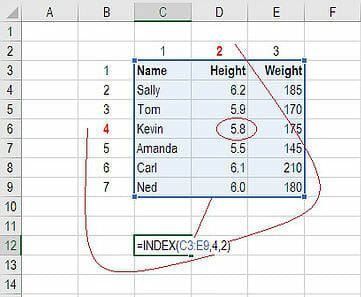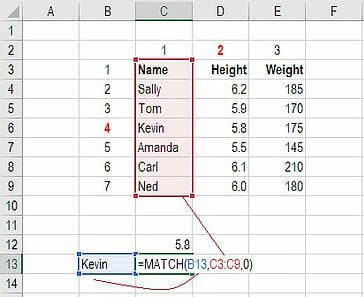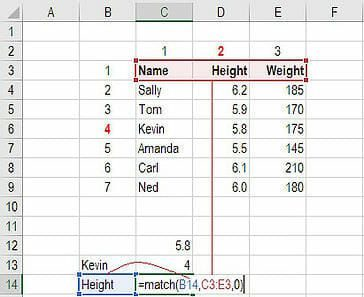This article may be out of date!

# Objective:

• Cross-reference data from multiple reports using Excel
• Using INDEX / MATCH to create custom reports
• How do I combine reports?

# Environment:

• Clio Manage
• Microsoft Excel

# Procedure:

### What is INDEX MATCH in Excel?

The INDEX MATCH formula is the combination of two functions in Excel: INDEX and MATCH.

• =INDEX() returns the value of a cell in a table based on the column and row number.
• =MATCH() returns the position of a cell in a row or column.

Combined, the two formulas can look up and return the value of a cell in a table based on vertical and horizontal criteria. For short, this is referred to as just the Index Match function.

This is especially useful in Clio, as it allows you to bring in data from other reports, so long as there is data in common on both reports (such as a "Name", "Matter Number", or "ID" field, allowing you to cross-reference.)

The basic formula structure goes like this:

`=INDEX(contacts.csv!\$H:\$H,MATCH(matters.csv!F2,contacts.csv!\$A:\$A,0))`
• contacts.csv!\$H:\$H = Locked range that contains the values you want to pull in
• matters.csv!F2 = Unlocked single Cell in the current report that contains the lookup data (eg. the Client's Name or Matter Number) that you're using to cross-reference.
• contacts.csv!\$A:\$A = Locked range that contains the list of values you're cross-referencing against (such as the "Client Name" or "Matter Number" column)

### #1 How to Use the INDEX Formula

Below is a table showing people’s name, height and weight. We want to use the INDEX formula to look up Kevin’s height… here is an example of how to do it.

1. Type “=INDEX(” and select the area of the table then add a comma
2. Type the row number for Kevin, which is “4” and add a comma
3. Type the column number for Height, which is “2” and close the bracket
4. The result is “5.8”### #2 How to Use the MATCH Formula

Sticking with the same example as above, let’s use MATCH to figure out what row Kevin is in.

1. Type “=MATCH(” and link to the cell containing “Kevin”… the name we want to look up
2. Select the all the cells in the Name column (including the “Name” header)
3. Type zero “0” for an exact match
4. The result is that Kevin is in row “4”Use MATCH again to figure out what column Height is in.